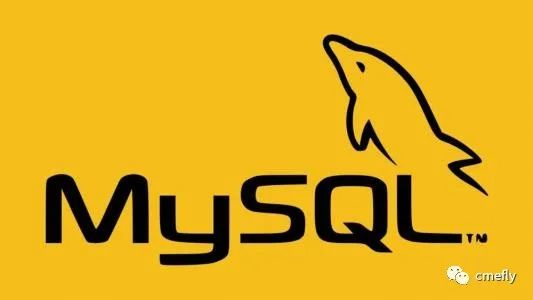问题背景：最近在用ThinkPHP 5开发项目的过程中，发现根据筛选条件做or查询的时候，连贯操作不可以使用where进行条件查询了。首先列出一个user数据表的信息：uidunamegrade(年级)class(班级)sex(性别)11号12122号11233号33244号42155号25166号16277号11188号23199号2211010号312数据表展现了10位同学的年级、班级、性别信息现在要查询数据为grade=1 or class= or sex=2在TP3中想要or查询条件可以为：$condition['grade'] = 1;$condition['class'] = 3;$condition['sex'] = 2;$condtion['_logic'] = 'OR';$list = M(‘user’)->where($condtion)->findall();然后在TP5中尝试用where去这么查询发现一直在报错，查了手册之后发现TP5取消了_logic作为查询方式，而是新增了whereOr方法，下面是TP5中查询方式User.phpnamespace app\index\controller;useapp\index\model\UserModel;classUser{public functionindex(){$condition['grade'] = 1;$condition['class'] = 3;$condition['sex'] = 2;$UserModel = newUserModel;$list =$UserModel->getlistwhereOr($condition);print_r($list);}}UserModel.phpnamespace app\index\model;useapp\common\model\CommonModel;usethink\Db;usethink\Model;class UserModel extendsCommonModel{public function__construct(){parent::__construct();}protected $name = 'User';public function getlistwhereOr($condition) {$list =Db::name($this->name)->whereOr($condition)->select();return$list;}}执行User.php 发现打印出来的数据就是我们要的筛选数据，总结：TP5相比TP3中更新了很多我们经常用到的查询方式，而且写法更人性化，需要经常的去整理查看这些新方法by as
展开全文• 有查询条件就查询，多个查询条件，只要有查询，就增加一个查询条件//类型if($sotype){$where['type']=$sotype;}//合作单位if($companyid){$where['hezuodanwei']=$companyid;}//关键词模糊查询$type是变量if($key){$... 有查询条件就查询，多个查询条件，只要有查询，就增加一个查询条件//类型if($sotype){$where['type'] =$sotype;}//合作单位if($companyid){$where['hezuodanwei'] = $companyid;}//关键词 模糊查询$type 是变量if($key){$where[$type] = ['like',"%".$key."%"];}$rs=Db::name('student')->where($where)->order('id desc')->limit($limit)->page($page)->select();$rs1=Db::name('student')->where($where)->select();$where['type'] =$sotype;$where['hezuodanwei'] =$companyid;$where["username"] = ['like',"%".$tag["kw"]."%"];//模糊查询$where[]=['exp','FIND_IN_SET(2,needID)'];例子：id in(1,5,8)$where['hezuodanwei'] =array('in','10,12');组成查询数组$wherewhere($where)引用：http://blog.csdn.net/u010447573/article/details/47420063Where 条件表达式格式为：$map['字段名'] = array('表达式', '操作条件');其中$map 是一个普通的数组变量，可以根据自己需求而命名。上述格式中的表达式实际是运算符的意义：ThinkPHP运算符 与 SQL运算符 对照表TP运算符SQL运算符例子实际查询条件eq=$map['id'] = array('eq',100);等效于：$map['id'] = 100;neq!=$map['id'] = array('neq',100);id != 100gt>$map['id'] = array('gt',100);id > 100egt>=$map['id'] = array('egt',100);id >= 100lt<$map['id'] = array('lt',100);id < 100elt<=$map['id'] = array('elt',100);id <= 100likelike$map = array('like','Admin%');username like 'Admin%'betweenbetween and$map['id'] = array('between','1,8');id BETWEEN 1 AND 8not betweennot between and$map['id'] = array('not between','1,8');id NOT BETWEEN 1 AND 8inin$map['id'] = array('in','1,5,8');id in(1,5,8)not innot in$map['id'] = array('not in','1,5,8');id not in(1,5,8)and(默认)and$map['id'] = array(array('gt',1),array('lt',10));(id > 1) AND (id < 10)oror$map['id'] = array(array('gt',3),array('lt',10), 'or');(id > 3) OR (id < 10)xor(异或)xor两个输入中只有一个是true时，结果为true，否则为false，例子略。1 xor 1 = 0exp综合表达式$map['id'] = array('exp','in(1,3,8)');$map['id'] = array('in','1,3,8');补充说明同 SQL 一样，ThinkPHP运算符不区分大小写，eq 与 EQ 一样。between、 in 条件支持字符串或者数组，即下面两种写法是等效的：$map['id'] = array('not in','1,5,8');$map['id']  = array('not in',array('1','5','8'));exp 表达式上表中的 exp 不是一个运算符，而是一个综合表达式以支持更复杂的条件设置。exp 的操作条件不会被当成字符串，可以使用任何 SQL 支持的语法，包括使用函数和字段名称。exp 不仅用于 where 条件，也可以用于数据更新，如：$Dao = M("Article");// 构建 save 的数据数组，文章点击数+1$data['id'] = 10;$data['counter'] = array('exp','counter+1');// 根据条件保存修改的数据$User->save($data);官方查询语法：https://www.kancloud.cn/manual/thinkphp5/135182查询表达式版本新增功能5.0.9比较运算增加闭包子查询支持5.0.4支持对同一个字段多次调用查询方法查询表达式支持大部分的SQL查询语法，也是ThinkPHP查询语言的精髓，查询表达式的使用格式：where('字段名','表达式','查询条件');whereOr('字段名','表达式','查询条件');表达式不分大小写，支持的查询表达式有下面几种，分别表示的含义是：表达式含义EQ、=等于(=)NEQ、<>不等于(<>)GT、>大于(>)EGT、>=大于等于(>=)LT、<小于(ELT、<=小于等于(<=)LIKE模糊查询[NOT] BETWEEN(不在)区间查询[NOT] IN(不在)IN 查询[NOT] NULL查询字段是否(不)是NULL[NOT] EXISTSEXISTS查询EXP表达式查询，支持SQL语法> time时间比较< time时间比较between time时间比较notbetween time时间比较表达式查询的用法示例如下：EQ ：等于(=)例如：where('id','eq',100);where('id','=',100);和下面的查询等效where('id',100);表示的查询条件就是 id = 100NEQ： 不等于(<>)例如：where('id','neq',100);where('id','<>',100);表示的查询条件就是 id <> 100GT：大于(>)例如：where('id','gt',100);where('id','>',100);表示的查询条件就是 id > 100EGT：大于等于(>=)例如：where('id','egt',100);where('id','>=',100);表示的查询条件就是 id >= 100LT：小于(例如：where('id','lt',100);where('id','表示的查询条件就是 id < 100ELT： 小于等于(<=)例如：where('id','elt',100);where('id','<=',100);表示的查询条件就是 id <= 100[NOT] LIKE： 同sql的LIKE例如：where('name','like','thinkphp%');查询条件就变成 name like 'thinkphp%'V5.0.5+版本开始，like查询支持使用数组where('name','like',['%think','php%'],'OR');[NOT] BETWEEN ：同sql的[not] between查询条件支持字符串或者数组，例如：where('id','between','1,8');和下面的等效：where('id','between',[1,8]);查询条件就变成 id BETWEEN 1 AND 8[NOT] IN： 同sql的[not] in查询条件支持字符串或者数组，例如：where('id','not in','1,5,8');和下面的等效：where('id','not in',[1,5,8]);查询条件就变成 id NOT IN (1,5, 8)[NOT] IN查询支持使用闭包方式[NOT] NULL ：查询字段是否(不)是Null，例如：where('name', null);where('title','null');where('name','not null');如果你需要查询一个字段的值为字符串null或者not null，应该使用：where('title','=', 'null');where('name','=', 'not null');EXP：表达式支持更复杂的查询情况 例如：where('id','in','1,3,8');可以改成：where('id','exp',' IN (1,3,8) ');exp查询的条件不会被当成字符串，所以后面的查询条件可以使用任何SQL支持的语法，包括使用函数和字段名称。 展开全文• 1.where(['arc.category_id'=&... 1]) 数组多条件设置查询 下方关联查询$articleList = Db::name("tp_art_rela_category") -&gt;alias("arc") -&gt;join('tp_article...
1.  where(['arc.category_id'=> $category_id ,'status' => 1]) 数组多条件设置查询 下方关联查询 $articleList = Db::name("tp_art_rela_category")
->alias("arc")
->join('tp_article a','a.art_id = arc.art_id','inner')
->where(['arc.category_id'=> $category_id ,'status' => 1]) ->order('a.art_id','desc') ->paginate(3);  展开全文• <pre><code>UPDATE participantes SET confirmado = 1 WHERE id = 19 AND id = 20 AND id = 21; </code></pre> <p>participantes -> Table <p>confirmado -> field of the table participantes. </div>mysql php • 今天 我们来聊聊日常工作中常会遇到的问题，mysql...二、通过操作的优化可通过 8 方法优化 Mysql 数据库：创建索引、复合索引、索引不会包含有 NULL 值的列、使用短索引、排序的索引问题、like 语句操作、不要在列...今天 我们来聊聊日常工作中常会遇到的问题，mysql的调优。可以从两方面入手：一、通过优化配置参数 如合适的 innodb 池大小，取消反向解析，合理的连接数，合理的超时时长，合理的相关 cache 等。二、通过操作的优化可通过 8 个方法优化 Mysql 数据库：创建索引、复合索引、索引不会包含有 NULL 值的列、使用短索引、排序的索引问题、like 语句操作、不要在列上进行运算、不使用 NOT IN 和<>操作1、创建索引 索引不是越多越好，每个表控制在 6 个索引以内。范围 where 条件的情况下，索引不起作用，比如 where value<1002、复合索引(联合索引)比如有一条语句是这样的：select * from users where name='陈五' and sex=1;我们创建了(name, sex, phone)的复合索引，那么其实相当于创建了(name, sex, phone)、(name, sex)、(name)三个索引，这被称为最佳左前缀特性。因此我们在创建复合索引时应该将最常用作限制条件的列放在最左边，依次递减。3、索引不会包含有 NULL 值的列只要列中包含有 NULL 值都将不会被包含在索引中，复合索引中只要有一列含有 NULL 值，那么这一列对于此复合索引就是无效的。所以我们在数据库设计时不要让字段的默认值为 NULL。4、使用短索引 对串列进行索引，如果可能应该指定一个前缀长度。例如，如果有一个 CHAR(255)的 列，如果在前 10 个或 20 个字符内，多数值是唯一的，那么就不要对整个列进行索引。短索引不仅可以提高查询速度而且可以节省磁盘空间和 I/O 操作。5、排序的索引问题 mysql 查询只使用一个索引，因此如果 where 子句中已经使用了索引的话，那么 order by 中的列是不会使用索引的。因此数据库默认排序可以符合要求的情况下不要使用排序操作；尽量不要包含多个列的排序，如果需要最好给这些列创建复合索引。6、like 语句操作 一般情况下不鼓励使用 like 操作，如果非使用不可，如何使用也是一个问题。like “%aaa%” 不会使用索引而 like “aaa%”可以使用索引。7、不要在列上进行运算 select * from users where YEAR(create_time) < 2020; 将在每个行上进行运算，这将导致索引失效而进行全表扫描，因此我们可以改成 select * from users where create_time < '2020-01-01' ;8、不使用 NOT IN 和<>操作 NOT IN 和<>操作都不会使用索引将进行全表扫描。NOT IN 可以 NOT EXISTS 代替，id<>10 则可使用 id>10 or id<10 来代替。注意：1、索引要建立在经常进行select操作的字段上这是因为，如果这些列很少用到，那么有无索引并不能明显改变查询速度。相反，由于增加了索引，反而降低了系统的维护速度和增大了空间需求。2、索引要建立在值比较唯一的字段上。3、对于那些定义为text、image和bit数据类型的列不应该增加索引。因为这些列的数据量要么相当大，要么取值很少。4、在where和join中出现的列需要建立索引。5、where的查询条件里有不等号(where column != …),mysql将无法使用索引。6、如果where字句的查询条件里使用了函数(如：where DAY(column)=…),mysql将无法使用索引。7、在join操作中(需要从多个数据表提取数据时)，mysql只有在主键和外键的数据类型相同时才能使用索引，否则及时建立了索引也不会使用。最后，多用 explain 和 profile 分析查询语句。  展开全文• 在查询时针对where条件中每个列找到满足值域的Term列表，再对应产生多个DocId列表； 再通过拉链合并算法，最终得到合并DocId之后的最大公共集合； 基于这个合并之后的DocId集合（即Pk集合），再回主表查询数据和... • 我这里将来解决如何在MySQL执行update，delete等需要查询的语句，最后限制where后跟多个条件的问题。比如，我现在在数据库当中有如下一张表。当我想要更新name为”louyujing”，type为1的string当中的内容，就可以...mysql • 我试图将多个参数添加到if语句中.我正在尝试检查两队之间的比赛是否是联赛.SELECT visitor AS school, home AS temp, vl.leagueid AS vleague, hl.leagueid AS hleague,if(vl.leagueid = hl.leagueid, 1, 0) AS ... • MySQL可以为多个列创建索引。一个索引可以包括15个列。对于某些列类型，可以索引列的前缀(参见7.4.3节，“列索引”)。多列索引可以视为包含通过连接索引列的值而创建的值的排序的数组。MySQL按这样的方式使用多列... • where 后边跟同一条件多值查询用 “ in （）‘’ SELECT * FROM sys_dept WHERE id IN ( 1253175466772705281, 1352263455977005058 ) 不同条件用 and SELECT * FROM sys_dept WHERE id = ...sql mysql • 如果记录中有两都是 唯一标识的 ，那是都where and 还是只写一比较快 ---- 一 • and I need to be able to make the difference between a fetch where number = 0 and number != 0 <pre><code>SELECT name, animal FROM users INNER JOIN pet ON users.id = pet.user WHERE number != 0...mysql php • products表如下：a WHERE后以and 接两个条件以及三个条件进行搜索：b 使用or来搜索多个条件c 下面这个有点意思，找出由vend_id 为1002或者1003生产的价格大于10的产品，这里需要使用and 和 or进行组合：但是从上面的... • select * from table where id=1 and uid=2 and (status=2 or status=3 or status=4); 转载于:https://www.cnblogs.com/houweijian/p/3314648.html • 上一节我们讲了 select 关键词的用法。...where: 告诉数据库，执行筛选操作筛选条件：指定筛选条件where 关键词具体要怎么用？我们仍以豆瓣 Top250 电影表为例来讲解。假如我们要把电影列成一张单子... • 多个查询条件，只要有查询，就增加一个查询条件 一、TP5.1版本 模糊查询$where[] = ['title','like',"%".$sotitle."%"];$map[] = ['name','like','think']; $map[] = ['status','=',1]; //时间查询$wheret2...
• 我最近在开发的一项目中，因为涉及到了表的复杂查询，需要在一sql里同时包含两处where查询条件，然后就百度不到了。。。后来通过自己查看mybatis plus的源码，才发现原来是用到了ParamAlias这属性。 where...
• two = n.id r.node_two = #{keyWord} OR n.model_name LIKE '%${keyWord}%' OR r.relation LIKE '%${keyWord}%' group by r.node_one,r.node_two having r.is_delete = 0 and r.node_one=#{nodeId1} 选择条件可以用...
• WHERE可以按多条件进行搜索。 products表如下： ...b 使用or来搜索多个条件 c 下面这个有点意思，找出由vend_id 为1002或者1003生产的价格大于10的产品，这里需要使用and 和 or进行组合：mysql 搜索
• .AsEnumerable()延迟执行，不会立即执行。... 下面的两实体类的多条件查询就是运用了先AsEnumerable，再ToList，这样可以直接执行一次，不然就去执行次ToList using (var db = new DB()) { ...
• I am experienced in R and new to Python ... I am trying to index a DataFrame to retrieve rows that meet a set of several logical conditions - much like the "where" statement of SQL.I know how to ...
• LEFT JOIN ON 后面跟多个条件Where的区别,如果认为LEFT JOIN的 On后面跟的条件和写在Where后面一样，那你就错了,测试LEFTJOIN 发现，ON后面跟了好几个条件
• 在《MySQL 常见语句加锁分析》一文中，我们详细讲解了 SQL 语句的加锁原理并具体分析了大部分的简单 SQL 语句，但是实际业务场景中 SQL 语句往往及其复杂，包含多个条件，此时就需要具体分析SQL 使用到的索引，并...
• 连接符链接多个条件 生成的sql语句 where条件也是用&链接的 会导致不能正确查询出数据 例如 .Where(it => it.A == A & it.B == B); 这个情况就查询不出数据 把&换成2个&&...
• [code="ruby"] scope :by_category, (lambda do |category_id| where(category_id: category_id) unless category_id.nil? ... scope :by_district, (lambda do |district_id| ... where(district...ruby rails...